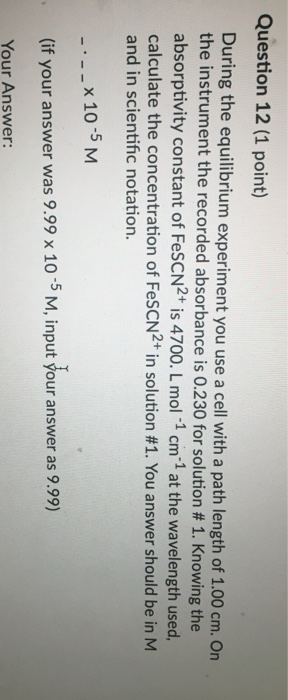# Question Question 12 (1 point) During the equilibrium experiment you use a cell with a path length of 1.00 cm. On the instrument the recorded absorbance is 0.230 for solution # 1. Knowing the absorptivity constant of FeSCN2+ is 4700. L mol-1 cm 1 at the wavelength used, calculate the concentration of FeSCN2+ in solution #1. You answer should be in M and in scientific notation. --- X 10 -5 M (if your answer was 9.99 x 10-5 M, input your answer as 9.99) Your Answer: